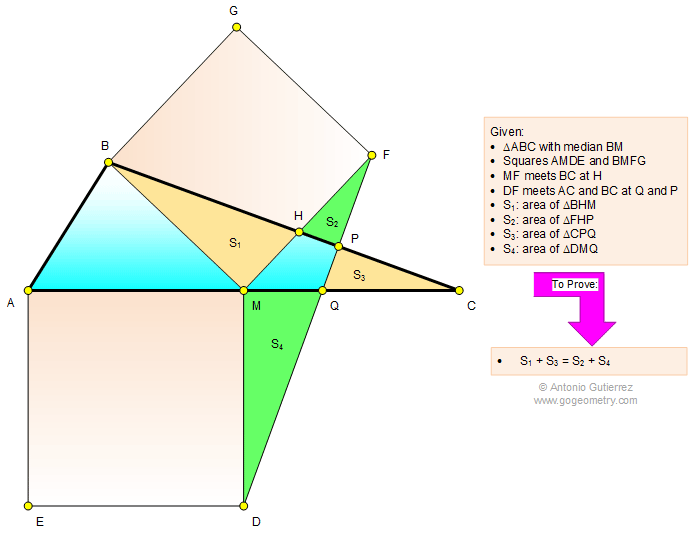# Geometry Problem 1124: Triangle, Median, Two Squares, Area. Level: High School, SAT Prep, Honors Geometry, College, Math Education

< PREVIOUS PROBLEM  |  NEXT PROBLEM >

 The figure below shows a triangle ABC with the median BM. AMDE and BMFG are squares so that MF meets BC at H and DF meets AC and BC at Q and P, respectively. If S1, S2, S3, and S4 are the areas of triangles BHM, FHP, CPQ, and DMQ, respectively, prove that S1 + S3 = S2 + S4.Home | Search | Geometry | Problems | All Problems | Open Problems | Visual Index | 10 Problems | 1121-1130 | Triangle | Median | Square | Triangle & Squares | Perpendicular lines | Area | Triangle area | Email | Solution / comment.Last updated: Jun 19, 2015 by Antonio Gutierrez.